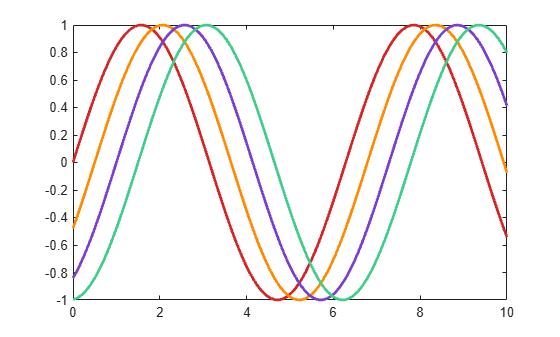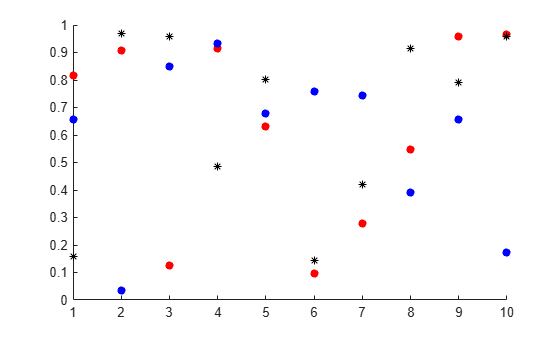# colororder

Set color order for visualizing multiple data series

## Syntax

``colororder(newcolors)``
``colororder(target,newcolors)``
``C = colororder``
``C = colororder(target)``

## Description

example

````colororder(newcolors)` sets the color order for the current figure. If a figure does not exist, MATLAB® creates a figure and sets the color order for that figure. When you set the color order for a figure, you set the color order for all the axes within that figure.```

example

````colororder(target,newcolors)` sets the color order for the target axes, figure, or chart instead of the current figure.```
````C = colororder` returns the color order matrix for the current figure.```

example

````C = colororder(target)` returns the color order matrix for the target figure, axes, or chart.```

## Examples

collapse all

Set the color order for the figure to four colors. Define an x-coordinate vector and four y-coordinate vectors. Then plot each set of coordinates.

```newcolors = [0.83 0.14 0.14 1.00 0.54 0.00 0.47 0.25 0.80 0.25 0.80 0.54]; colororder(newcolors) % Define coordinates x = linspace(0,10); y1 = sin(x); y2 = sin(x-0.5); y3 = sin(x-1); y4 = sin(x-1.5); % Plot coordinates plot(x,y1,'LineWidth',2) hold on plot(x,y2,'LineWidth',2) plot(x,y3,'LineWidth',2) plot(x,y4,'LineWidth',2) hold off```Plot seven concentric quarter circles.

```hold on for r=1:7 x = linspace(0,r,500); y = sqrt(r.^2-x.^2); plot(x,y,'LineWidth',15) end```Change the color order to seven hexadecimal color codes.

```newcolors = {'#F00','#F80','#FF0','#0B0','#00F','#50F','#A0F'}; colororder(newcolors)```Display three series of bars. Then set the color order to blue, purple, and gray.

```bar([10 20 30; 25 35 45; 30 40 52]) newcolors = [0 0.5 1; 0.5 0 1; 0.7 0.7 0.7]; colororder(newcolors)```Setting the color order for the figure before calling `yyaxis` sets the color for each y-axis. The left side uses the first color, and the right side uses the second color. If you specify more than two colors, the additional colors are not used by either side.

Define `newcolors` as a matrix containing two RGB triplets. Set the color order for the figure, and plot two lines against the left side. Then plot two lines against the right side.

```newcolors = [0.40 0.30 0.90; 0.50 0.65 0.15]; colororder(newcolors) % Left side yyaxis left plot([1 2; 3 4]) % Right side yyaxis right plot([4 3; 2 1])```Setting the color order for the figure after calling `yyaxis` sets the color for the active side.

Activate the left y-axis and plot three lines. Set the line style order to one solid line and change the y-axis color to blue. Then set the color order to three shades of blue.

```% Left side yyaxis left plot([1 2 3; 4 5 6]) ax = gca; ax.LineStyleOrder = '-'; ax.YColor = 'blue'; leftcolors = [0 0 1; 0 0.50 1; 0 0.80 1]; colororder(leftcolors)```Activate the right y-axis and plot two lines. Change the y-axis color to black. Then set the color order to black.

```% Right side yyaxis right plot([4 3; 2 1]) ax.YColor = 'black'; colororder('black')```When you call a plotting function with a color argument, the plotting function uses that color instead of the next color in the color order.

Set the color order of the figure to red, magenta, and blue. Call the `scatter` function to plot a series of scattered points. Then plot a second series of points, and specify the markers as black asterisks.

```newcolors = {'red','magenta','blue'}; colororder(newcolors) scatter(1:10,rand(1,10),'filled') hold on scatter(1:10,rand(1,10),'*k')```Plot a third series of points without specifying the marker color. Notice that this series uses the third color in the color order, which is blue.

```scatter(1:10,rand(1,10),'filled') hold off```Create a tiled chart layout and plot three lines in the first tile.

```tiledlayout('flow') nexttile plot([1 2 3; 4 5 6],'LineWidth',2)```Call the `nexttile` function with a return argument to get the axes object for the second tile. Plot three lines in the second tile. Then get the color order matrix for the axes and return the output in `C`. Change the first color in `C` to purple, and set the axes color order to the modified `C` matrix.

```ax = nexttile; plot(ax,[4 5 6; 1 2 3],'LineWidth',2) C = colororder(ax); C(1,:) = [0.5 0 1]; colororder(ax,C)```## Input Arguments

collapse all

New colors, specified as a matrix of RGB triplets, an array of color names, or `'default'`.

#### Matrix of RGB Triplets

Specify an m-by-3 matrix, where each row is an RGB triplet. An RGB triplet is a three-element vector containing the intensities of the red, green, and blue components of a color. The intensities must be in the range `[0,1]`. For example, this matrix defines the new colors as blue, dark green, and orange:

```newcolors = [1.0 0.0 0.0 0.0 0.4 0.0 1.0 0.5 0.0];```

#### Array of Color Names or Hexadecimal Color Codes

Specify any combination of color names, short names, or hexadecimal color codes.

• To specify one color, set `newcolors` to a character vector or a string scalar. For example, `newcolors = 'red'` specifies red as the only color in the color order.

• To specify multiple colors, set `newcolors` to a cell array of character vectors or a string array. For example, ```newcolors = {'red','green','blue'}``` specifies red, green, and blue as the colors.

A hexadecimal color code starts with a hash symbol (`#`) followed by three or six hexadecimal digits, which can range from `0` to `F`. The values are not case sensitive. Thus, the color codes `'#FF8800'`, `'#ff8800'`, `'#F80'`, and `'#f80'` are equivalent.

This table lists the color names and short names with the equivalent RGB triplets and hexadecimal color codes.

Color NameShort NameRGB TripletHexadecimal Color CodeAppearance
`'red'``'r'``[1 0 0]``'#FF0000'``'green'``'g'``[0 1 0]``'#00FF00'``'blue'``'b'``[0 0 1]``'#0000FF'``'cyan'` `'c'``[0 1 1]``'#00FFFF'``'magenta'``'m'``[1 0 1]``'#FF00FF'``'yellow'``'y'``[1 1 0]``'#FFFF00'``'black'``'k'``[0 0 0]``'#000000'``'white'``'w'``[1 1 1]``'#FFFFFF'`#### Default Colors

Specify `'default'` to set the color order to the seven default colors. This option is useful for resetting the color order after you temporarily change it. Here are the RGB triplets and hexadecimal color codes for the default colors.

`[0 0.4470 0.7410]``'#0072BD'``[0.8500 0.3250 0.0980]``'#D95319'``[0.9290 0.6940 0.1250]``'#EDB120'``[0.4940 0.1840 0.5560]``'#7E2F8E'``[0.4660 0.6740 0.1880]``'#77AC30'``[0.3010 0.7450 0.9330]``'#4DBEEE'``[0.6350 0.0780 0.1840]``'#A2142F'`Data Types: `single` | `double` | `char` | `cell` | `string`

Target, specified as one of these values:

collapse all

### Color Order

The color order controls the set of colors that MATLAB uses for plotting multiple data series within an axes. Graphics objects such as `Line`, `Scatter`, and `Bar` objects are assigned colors according to their order of creation.

The colors are stored as a matrix in the `ColorOrder` property of the axes. Calling the `colororder` function replaces the matrix.

## Tips

• When you set the color order for a figure, the colors persist when you call a plotting function. However, if you pass an axes object to the `colororder` function, you must first call `hold on` to make the colors persist when you call a plotting function.

• If you set the `ColorOrderIndex` or `LineStyleOrderIndex` property on the axes, the new color order does not affect existing plots. The new colors take effect only after you call ```hold on``` and then call a plotting function.

## Version History

Introduced in R2019b